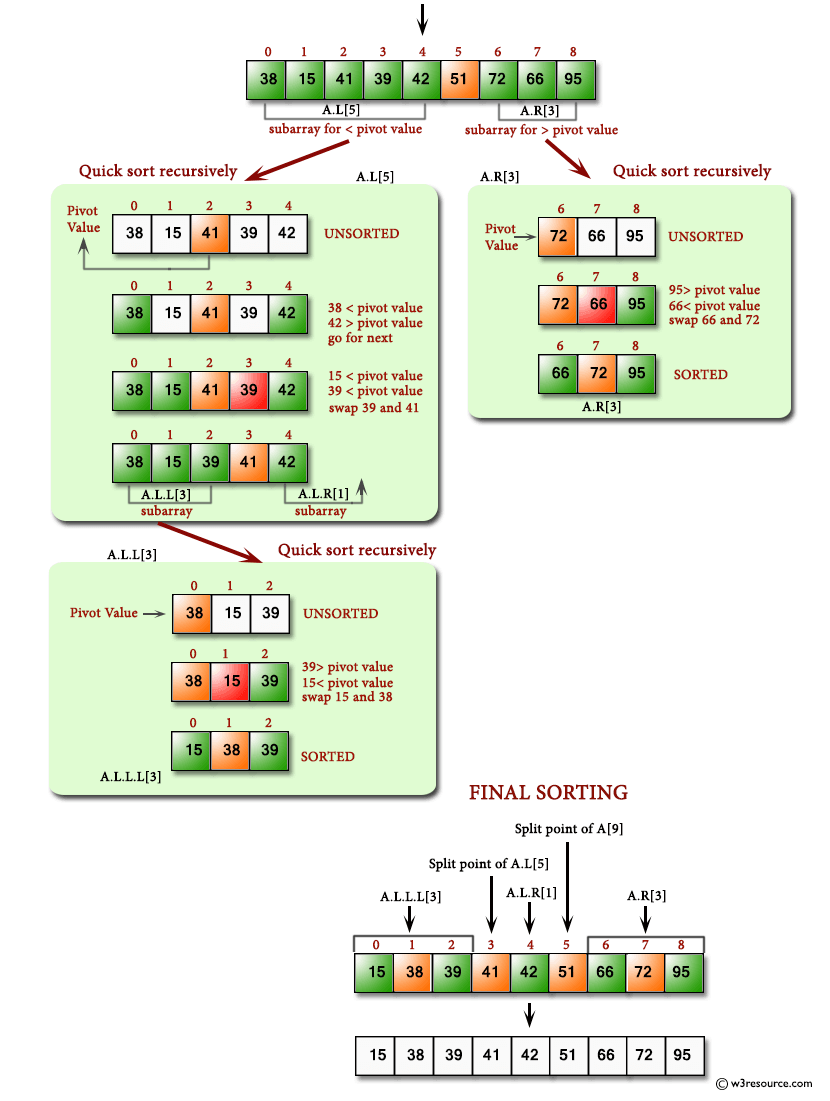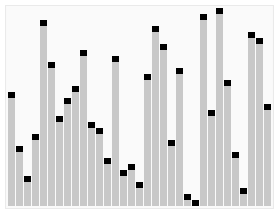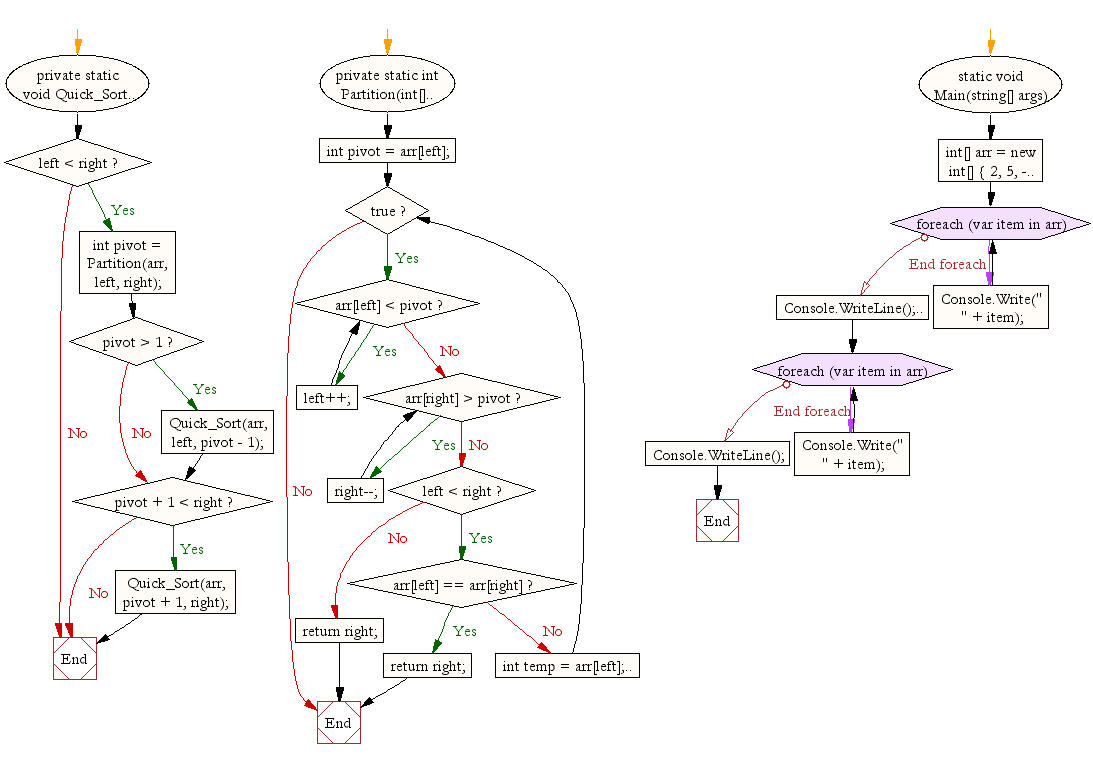﻿ C# Sharp exercises: Quick sort - w3resource# C# Sharp Searching and Sorting Algorithm Exercises: Quick sort

## C# Sharp Searching and Sorting Algorithm: Exercise-9 with Solution

Write a C# Sharp program to sort a list of elements using Quick sort.

Quick sort is a comparison sort, meaning that it can sort items of any type for which a "less-than" relation (formally, a total order) is defined.

Pictorial presentation - Quick Sort algorithm :Animated visualization of the quicksort algorithm. The horizontal lines are pivot values.

Animation credits : RolandH

Sample Solution:-

C# Sharp Code:

``````using System;
using System.Collections.Generic;
using System.Linq;
using System.Text;

namespace Quick_Sort
{
class Program
{
private static void Quick_Sort(int[] arr, int left, int right)
{
if (left < right)
{
int pivot = Partition(arr, left, right);

if (pivot > 1) {
Quick_Sort(arr, left, pivot - 1);
}
if (pivot + 1 < right) {
Quick_Sort(arr, pivot + 1, right);
}
}

}

private static int Partition(int[] arr, int left, int right)
{
int pivot = arr[left];
while (true)
{

while (arr[left] < pivot)
{
left++;
}

while (arr[right] > pivot)
{
right--;
}

if (left < right)
{
if (arr[left] == arr[right]) return right;

int temp = arr[left];
arr[left] = arr[right];
arr[right] = temp;

}
else
{
return right;
}
}
}
static void Main(string[] args)
{
int[] arr = new int[] { 2, 5, -4, 11, 0, 18, 22, 67, 51, 6 };

Console.WriteLine("Original array : ");
foreach (var item in arr)
{
Console.Write(" " + item);
}
Console.WriteLine();

Quick_Sort(arr, 0, arr.Length-1);

Console.WriteLine();
Console.WriteLine("Sorted array : ");

foreach (var item in arr)
{
Console.Write(" " + item);
}
Console.WriteLine();
}
}
}
```
```

Sample Output:

```Original array :
2 5 -4 11 0 18 22 67 51 6

Sorted array :
-4 0 2 5 6 11 18 22 51 67
```

Flowchart:C# Sharp Code Editor: